## MECHANISM OF PEAK DISCHARGE ENHANCEMENT OF CASCADE DAM BREAK FLOODS 1)

Huang Can, Liu Qingquan, Wang Xiaoliang,2)

Department of Mechanics, Beijing Institute of Technology, Beijing 100081, China

 基金资助: 1)国家自然科学基金.  11872117国家自然科学基金.  11602278重点研发项目.  2018YFC1505504北京理工大学青年教师学术启动计划

Received: 2020-02-15   Accepted: 2020-04-24   Online: 2020-05-18Abstract

Keywords： cascade dam ; dam break flow ; enhance ; shallow water flow

Huang Can, Liu Qingquan, Wang Xiaoliang. MECHANISM OF PEAK DISCHARGE ENHANCEMENT OF CASCADE DAM BREAK FLOODS 1). Chinese Journal of Theoretical and Applied Mechanics[J], 2020, 52(3): 645-655 DOI:10.6052/0459-1879-20-044

## 1 控制方程与数值方法

### 1.1 控制方程

$\frac{\partial h}{\partial t}+\frac{\partial hu}{\partial x}=0$
$\frac{\partial hu}{\partial t}+\frac{\partial }{\partial x}\lt(hu^2+\frac{1}{2}gh^2)=-gh\frac{\partial b}{\partial x}$

### 1.2 数值方法

$\frac{\partial U}{\partial t}+\frac{\partial F}{\partial x}=s(U)$

$\frac{d}{{d}t}U_j =-\frac{1}{\Delta x}\lt(F_{j+{1}/{2}} -F_{j-{1}/{2}})+S_i +S_{ci}$

$F_{j+{1}/{2}} =f(U_{j+({1}/{2})-} ,U_{j+({1}/{2})+} )$

$S_j =S_{j+(1/2)-} +S_{j+(1/2)+}$
$S_{cj} =(0,g({h_{j,l} +h_{j,r}})(b_{i,l} -b_{i,r} )/2)$

$\begin{eqnarray*} &&S_{j+(1/2)-}=\lt(0,\frac{1}{2}gh_{j+(1/2)-}^2 -\frac{1}{2}gh_{i,r}^2 )^{T}\\ &&S_{j-(1/2)+}=\lt(0,\frac{1}{2}gh_{j,l}^2 -\frac{1}{2}gh_{j-(1/2)+}^2)^{T} \end{eqnarray*}$

1.3.1 溃坝问题简单波解

### 图1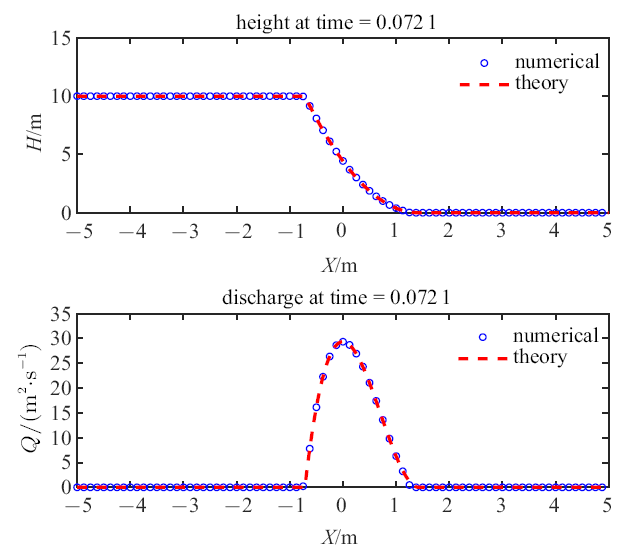Fig.1   Height and discharge distribution of dam break flow at 0.0721 s, the red dashed line and blue symbols are analytical and numerical solutions respectively, where $H$ is water depth, and $Q$ is discharge

1.3.2 Well-Balance行为

### 图2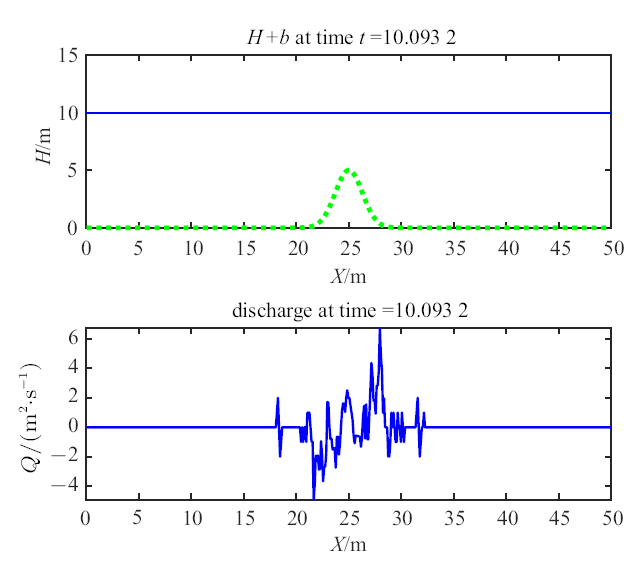Fig.2   Verification for Well-Balance behavior over a bump denoted by red dashed line, where $H+b$ is free surface elevation, and $Q$ is discharge

## 2 梯级坝溃决洪水演进

### 图3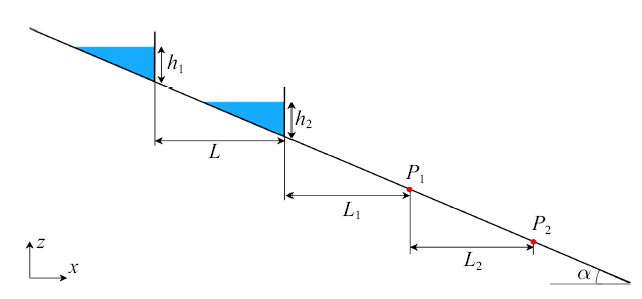Fig.3   Cascade dam-break model and detection points $P_1$ and $P_2$

### 图4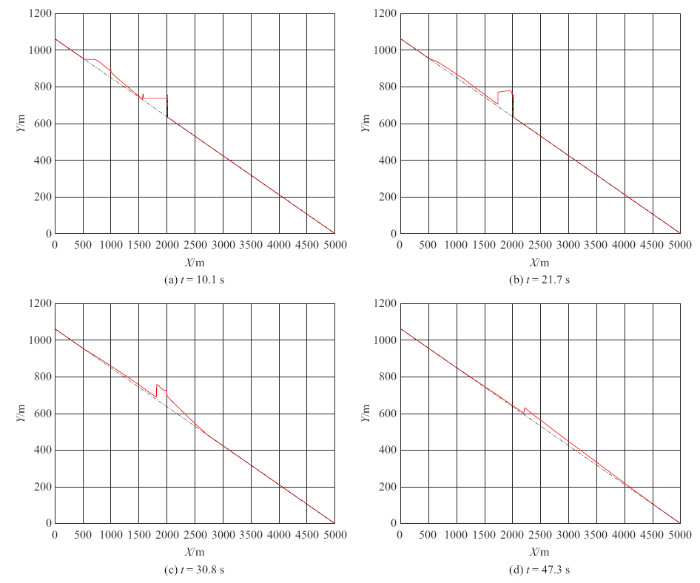Fig.4   Water elevation distribution at four typical times of cascade dam break flow

### 图5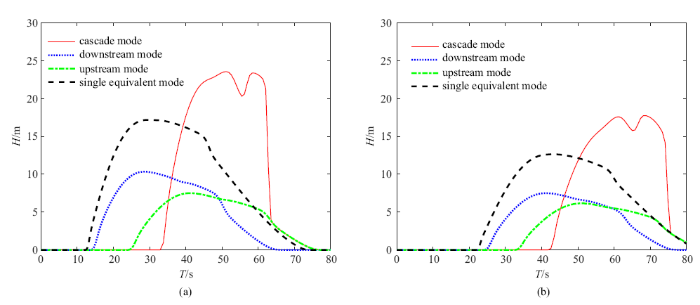Fig.5   Flood elevation evolution at detection points $P_1$ (a) and $P_2$ (b) for four dam break modes: cascade dam break mode, single downstream dam break mode, single upstream dam break mode, and single downstream dam break with full water mode

## 3 梯级坝溃决洪峰增强机制

### 图6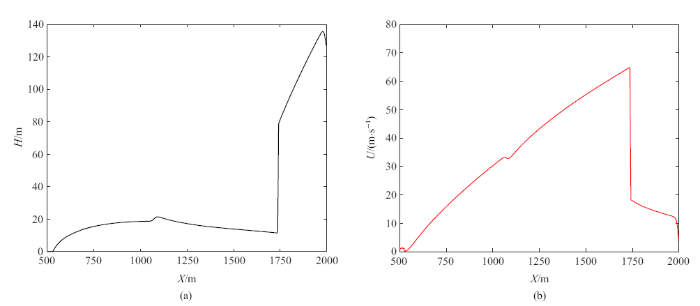Fig.6   Depth (a) and velocity (b) distribution of flood when downstream dam breaks

### 图7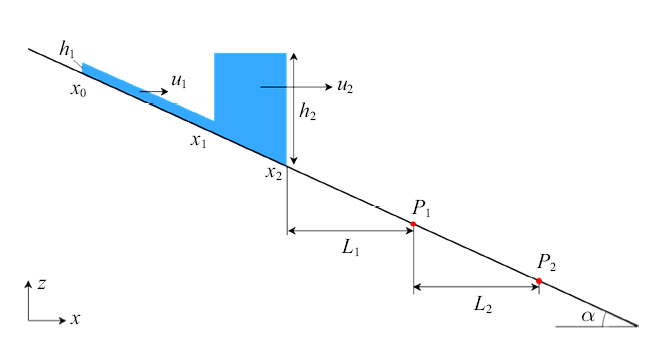Fig.7   Water tower model of cascade dam break flow

### 图8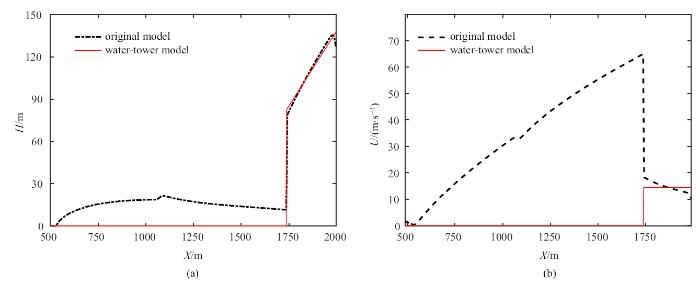Fig.8   Initial water depth (a) and velocity (b) of water tower model for cascade dam break flow

### 图9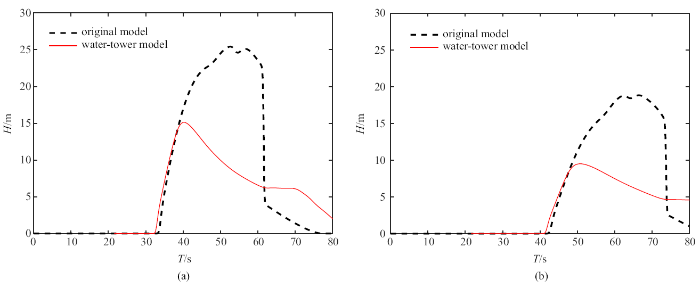Fig.9   Prediction of flood level at detection positions $P_1$ (a) and $P_2$ (b) of cascade dam break flow by water tower model, dashed line is shallow water flow model prediction, and solid line represents water tower model prediction

### 图10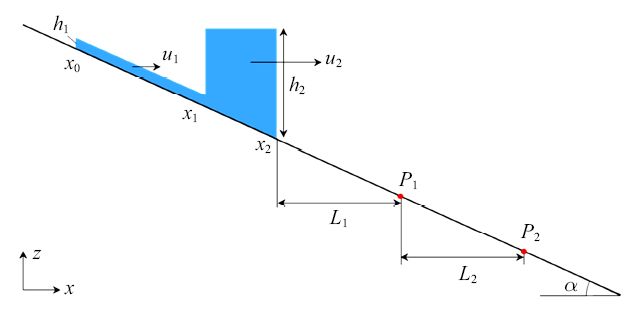Fig.10   Jet-water tower model for cascade dam break flow

### 图11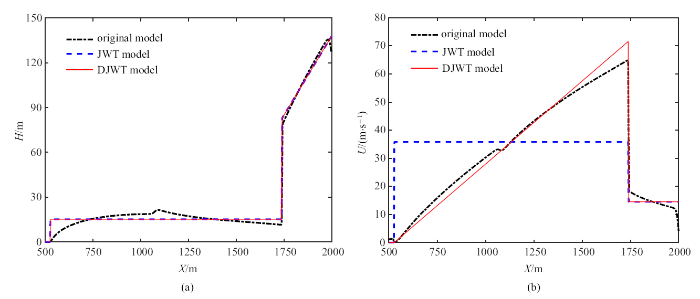Fig.11   Initial water depth (a) and velocity (b) of jet-water tower model for cascade dam break flow, blue dashed line, red solid line and black dash dot line represent water depth and velocity of jet-water tower model (JWT), distributed jet-water tower model (DJWT), and original shallow water model, respectively

### 图12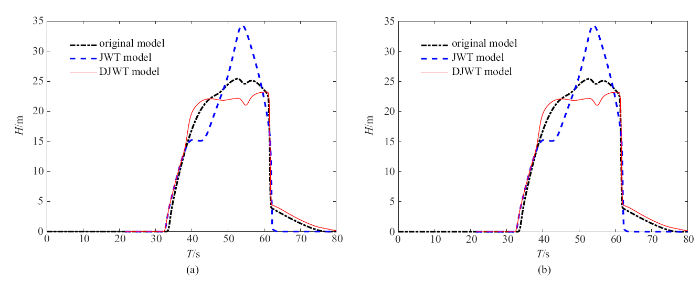Fig.12   Prediction of flood level at detection positions $P_1$ (a) and $P_2$ (b) of cascade dam break flow by jet-water tower model (JWT) in blue dashed line and distributed jet-water tower model (DJWT) in red solid line, and black dash dot line is the shallow water model solution

## 4 分布式"射流-水塔"模型应用

Table 1  Peak flood elevation prediction of real-scale cascade dam break flow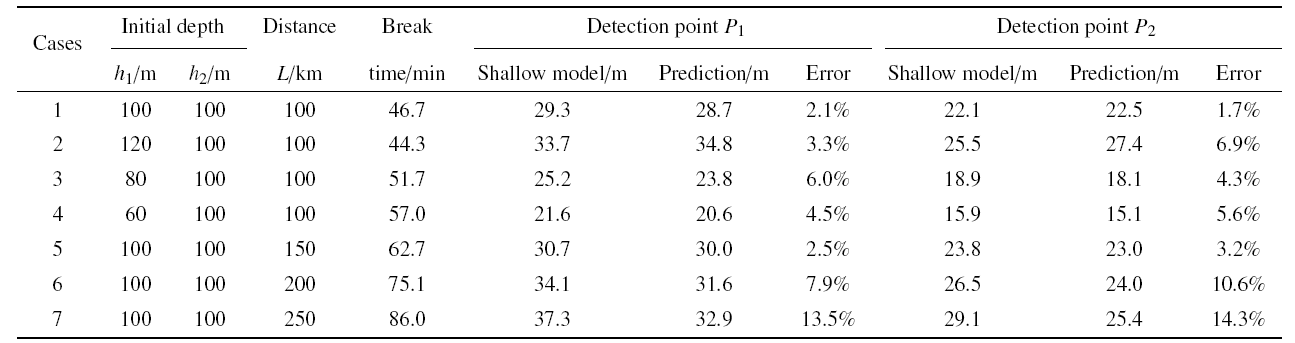Table 2  Peak flood discharge prediction of real-scale cascade dam break flow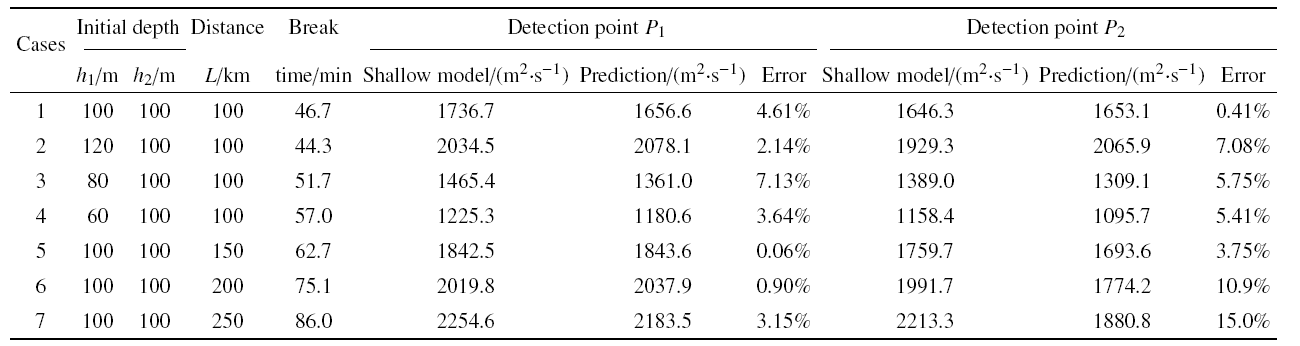## 5 结论

(1) 梯级溃决的洪水过程存在增强机制, 下游洪峰不仅超过单坝溃决洪峰, 而且超过两个单坝溃决的峰值之和, 以及所谓等体积溃决模式的洪峰.

(2) 梯级溃决的洪峰增强由射流水塔机制控制, 上游溃决诱发的洪水不仅增大下游水库的质量和动量, 形成"水塔", 而且在尾部残留一个动量较大的射流, 稳定和维持下游溃决后"水塔"的质量和动量.

(3) 以梯级溃决洪水演进增强的射流-水塔机制为基础, 建立了一个等效的射流水塔单坝溃决等效模型, 预测结果基本能够反映梯级溃决下游洪峰演化过程, 对实际百公里量级间距的梯级溃决洪峰过程预测良好.

/

 〈〉